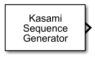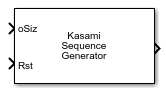# Kasami Sequence Generator

Generate Kasami sequence from set of Kasami sequences

• Library:
• Communications Toolbox / Comm Sources / Sequence Generators

•## Description

The Kasami Sequence Generator block generates a sequence from a set of Kasami sequences. The Kasami sequences are a set of sequences that have good cross-correlation properties. For more information, see Kasami Sequences.

These icons show the block with `oSiz`, `Ref` and `Rst` ports enabled.## Ports

### Input

expand all

Current output size, specified as a scalar or a vector of the form [n,1], where n is the number of elements in the output sequence.

Example: `[10 1]` a specifies a current output column vector of size 10-by-1.

#### Dependencies

To enable this port, set the Maximum output size source parameter to `Dialog parameter`.

Data Types: `double`

Reference input signal, specified as a scalar, or a column vector.

#### Dependencies

To enable this port, set the Maximum output size source parameter to `Inherit from reference input`.

Data Types: `double`

Reset signal, specified as a scalar or vector, depending on the output size.

• When the output size is variable, specify this port as a scalar.

• Otherwise, specify this port as a scalar or a column vector of length equal to the Samples per frame parameter value.

The output signal resets for nonzero Rst input values. For more information, see Reset Behavior.

#### Dependencies

To enable this port, select the Reset on nonzero input parameter.

Data Types: `double`

### Output

expand all

Output signal, returned as a binary-valued column vector. At least one element of the Initial states parameter vector must be nonzero for the block to generate a nonzero sequence.

Data Types: `double`

## Parameters

expand all

Specify the generator polynomial, which determines the connections in the shift register that generates the sequence, as one of these options.

• A polynomial character vector or string scalar that includes the number `1` (for example, ```'z^4 + z + 1'```). For more information, see Representation of Polynomials in Communications Toolbox.

• A binary-valued row vector that lists the coefficients of a polynomial in order of descending powers. The first and last entries must be `1`. The length of this vector must be one more than the degree of the generator polynomial.

• An integer-valued row vector containing the exponents of the nonzero terms of a polynomial in order of descending powers. The last entry must be `0`.

For example, `'z^8 + z^2 + 1'`, ```[1 0 0 0 0 0 1 0 1]```, and `[8 2 0]` represent the same polynomial p(z) = z8 + z2 + 1.

The initial states of the shift register generate the sequence. If you specify a binary-valued row vector, the length must equal the degree of the generator polynomial specified by the Generator polynomial parameter. If you specify a binary-valued scalar, the block expands the scalar to a row vector of length equal to the degree of the generator polynomial. All entries of the resulting vector equal the scalar.

Specify an integer or vector of the form [k m] to select a Kasami sequence of interest from the set of possible sequences. Two classes of Kasami sequences exist: those obtained from a small set and those obtained from a large set. You can choose a Kasami sequence from the small set by setting this parameter to an integer in the range [0, 2n/2–2]. You can choose a sequence from the large set by setting this parameter to a vector of the form [k m]. k must be an integer in the range [–2, 2n–2], and m must be an integer in the range [–1, 2n/2–2]. For more information, see Sequence Index.

Specify the offset of the Kasami sequence from the initial time.

You can use an external signal to reset the values of the internal shift register to the initial state by selecting the Reset on nonzero input parameter. This selection creates an input port for the external signal in this block. The way the block resets the internal shift register depends on whether its output signal and reset signal are sample-based or frame-based. For an example, see Reset Behavior.

Select this parameter to enable variable-length output sequences during simulation. When you clear this parameter, the block outputs fixed-length sequences. When you select this parameter, the block can output variable-length sequences. For information about variable-size signals, see Variable-Size Signal Basics (Simulink).

Select how to specify the maximum sequence output size.

• `Dialog parameter` — Select this value to configure the block to use the Maximum output size parameter setting as the maximum permitted output sequence length. The oSiz input port specifies the current size of the output signal, and the block output inherits the sample time from the input signal. The input value of oSiz must be less than or equal to the Maximum output size parameter.

• `Inherit from reference port` — Select this value to enable the Ref input port and configure the block to inherit the sample time, maximum size, and current output size from the variable-sized signal at the Ref input port. These set the maximum permitted output sequence length.

#### Dependencies

To enable this parameter, select Output variable-size signals.

Specify the maximum output size for the block. n is a positive scalar.

Example: `[10 1]` specifies a 10-by-1 maximum size for the output signal.

#### Dependencies

To enable this parameter, select Output variable-size signals and set Maximum output size source to ```Dialog parameter```.

Data Types: `double`

Positive scalars specify the time in seconds between each sample of the output signal. If you set the Sample time to `-1`, the output signal inherits the sample time from downstream. For information on the relationship between the Sample time and Samples per frame parameters, see Sample Timing.

#### Dependencies

To enable this parameter clear Output variable-size signals.

Specify the number of samples per frame in one channel of the output data. For information on the relationship between the Sample time and Samples per frame parameters, see Sample Timing.

Select this parameter to enable the Rst input port. Use that port to specify an input signal that resets the internal shift registers to the original values of the Initial states parameter value.

Specify the output data type of the block.

## Block Characteristics

 Data Types `Boolean` | `double` Multidimensional Signals `no` Variable-Size Signals `yes`